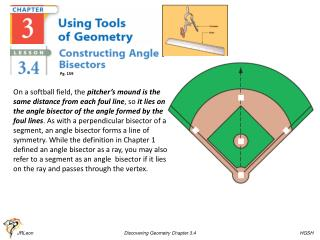DownloadDownload PresentationPg. 159

# Pg. 159

Télécharger la présentation## Pg. 159

- - - - - - - - - - - - - - - - - - - - - - - - - - - E N D - - - - - - - - - - - - - - - - - - - - - - - - - - -
##### Presentation Transcript

1. Pg. 159 On a softball field, the pitcher’s mound is the same distance from each foul line, so it lies on the angle bisector of the angle formed by the foul lines. As with a perpendicular bisector of a segment, an angle bisector forms a line of symmetry. While the definition in Chapter 1 defined an angle bisector as a ray, you may also refer to a segment as an angle bisector if it lies on the ray and passes through the vertex. JRLeon Discovering Geometry Chapter 3.4 HGSH

2. Draw an acute angle (about 60°) for this investigation. On patty paper, draw a large-scale angle. Label it PQR. Fold your patty paper so that QP and QR coincide. Crease the fold. Unfold your patty paper. Draw a ray with endpoint Q along the crease. Does the ray bisect PQR? How can you tell? Repeat Steps 1–3 with an obtuse angle. Do you use different methods for finding the bisectors of different kinds of angles? A Place a point on your angle bisector. Label it A. Compare the distances from A to each of the two sides. Remember that “distance” means shortest distance! Copy and complete the conjecture. JRLeon Discovering Geometry Chapter 3.4 HGSH

3. In this investigation, you will find a method for bisecting an angle using a compass and straightedge. Links: Angle Bisector Construct a 30° Angle Construct a 45°Angle Construct a 60° Angle Construct a 90° Angle http://www.mathopenref.com JRLeon Discovering Geometry Chapter 3.4 HGSH

4. JRLeon Discovering Geometry Chapter 3.5 HGSH

5. Constructing Parallel Lines with Compass Link: Constructing Parallel Lines JRLeon Discovering Geometry Chapter 3.5 HGSH

6. If two lines are parallel, how do their slopes compare? If two lines are perpendicular, how do their slopes compare? Perpendicular Slope Property In a coordinate plane, two nonvertical lines are perpendicular if and only if their slopes are opposite reciprocals of each other. Parallel Slope Property In a coordinate plane, two distinct lines are parallel if and only if their slopes are equal, or they are both vertical lines. JRLeon Discovering Geometry Chapter 3.5 HGSH

7. JRLeon Discovering Geometry Chapter 3.5 HGSH

8. Classwork/Homework: Lesson 3.4 Pages 160-161 Problems 1 thru 8 Lesson 3.5 Pages 164 Problems 1 thru 5 JRLeon Discovering Geometry Chapter 3.5 HGSH

9. JRLeon Discovering Geometry Chapter 3.5 HGSH

10. JRLeon Discovering Geometry Chapter 3.5 HGSH

11. JRLeon Discovering Geometry Chapter 3.5 HGSH